## Analogy

#### Analogy

Direction: Select the related figure from the given alternatives.

1. Find the question mark ? figure from answer figure.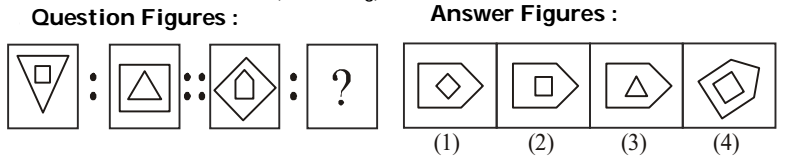1. From first figure to second figure both the designs interchange positions and one design is inverted.

##### Correct Option: A

From first figure to second figure both the designs interchange positions and one design is inverted.

1. Find the question mark ? figure from answer figure.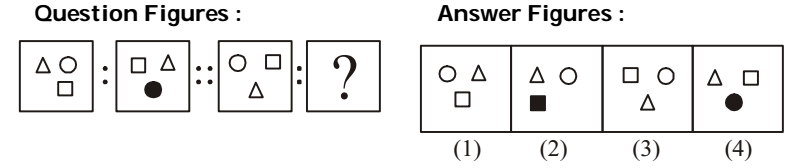1. From first figure to second figure the designs move in clockwise direction and the design which comes at the bottom position gets shaded.

##### Correct Option: B

From first figure to second figure the designs move in clockwise direction and the design which comes at the bottom position gets shaded.

1. Find the question mark ? figure from answer figure.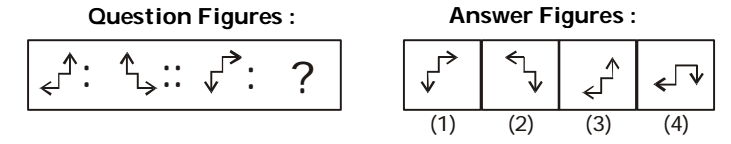1. The second figure is the mirror image of the first figure.

##### Correct Option: B

The second figure is the mirror image of the first figure.

1. Find the question mark ? figure from answer figure.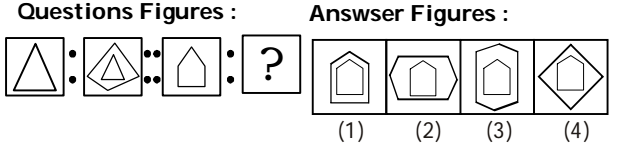1. From first figure to second figure the main design is enclosed by a new design have one more side than the main design.

##### Correct Option: C

From first figure to second figure the main design is enclosed by a new design have one more side than the main design.

1. Find the question mark ? figure from answer figure.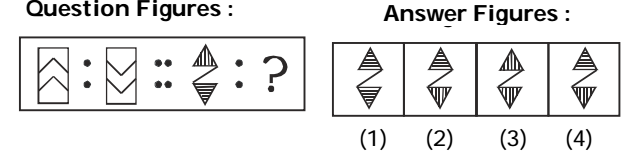1. From Problem Figure (1) to (2) the design is inverted vertically. In other words, the second figure is the water image of the first figure.

##### Correct Option: D

From Problem Figure (1) to (2) the design is inverted vertically. In other words, the second figure is the water image of the first figure.